# Formula Errors in Excel and Solutions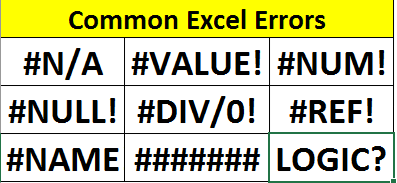"Errors are an opportunity to improve". In any task or system, errors occur. Excel is no exception. While trying to do something with a formula, you will encounter various types of excel errors. These errors can make your formula/dashboard/reports absolute waste. And if you don't know why a specific type of error has occurred, you may have to spend hours to resolve them.

While working on excel, I have encountered many excel errors. With some struggle and google searches, I worked around those errors. In this article, I will explain some common and annoying excel errors that occur in Excel. We will discuss why these errors occur and how to solve them.

What are Excel Formula Errors
While applying a formula that results in an excel defined errors (#NA, #VALUE, #NAME etc.) is called excel formula errors. These errors are caught by excel and printed on the sheets. Reasons for these errors can be, unavailable values, incorrect types of arguments, division by 0, etc. They are easy to catch and fix.

Logical errors are not caught by Excel and they are most difficult to fix. Inconsistency in data, wrong data entry, human errors etc. are a common reasons behind these errors. They can be fixed too but they take time and effort. It is better to prepare your data perfectly before doing operation on them.
Catching Excel Formula Errors:

There is some dedicated functions in excel to catch and handle a specific type of errors ( like ISNA function). But ISERROR function and IFERROR function are two functions that can catch any kind of Excel Error (except logical).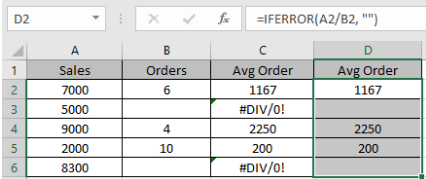Excel #NA Error
#NA error occurs in excel when a value is not found. It simply means NOT AVAILABLE. #NA error is often encountered with Excel VLOOKUP function.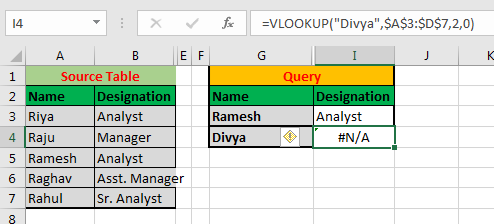In above image, we are getting #NA error when we look for "Divya" in the A column. This is because "Divya is not in the list.

Solving #NA Error

If you are sure that the lookup value must exist in the lookup list then the first thing you should do is check the lookup value. Check if the lookup values are correctly spelled. If not then correct it.

Secondly, you can do a partial match with VLOOKUP or any lookup function. If you are sure that some parts of the text must match then use this.
If you are not sure that value exists or not then it can be used to check if a value exists in the list or not. In the image above, we can say that Divya is not on the list.

If you want to catch the #NA error and print or do something else instead of printing #NA error then you can use Excel ISNA function. ISNA function returns TRUE if a function returns #NA error. Using this, we can avoid #NA error. ISNA works amazingly with the VLOOKUP function. Check it out here.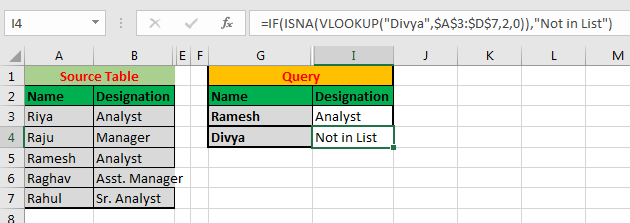Excel #VALUE Error
The #VALUE occurs when the supplied argument is not of a supported type. For example, if you try to add two texts using arithmetic plus operator (+), you will get a #VALUE error. The same thing will happen if you try to get a year of an invalid date format using the YEAR function.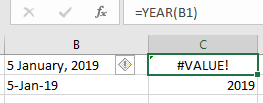How to fix a #VALUE error?

First, confirm the data type you are referring to. If your function requires a number, then make sure that you refer to a number. If a number is formatted as text then use the VALUE function to convert them into a number. If a function requires text (like DATEVALUE function) and you referring to a number or date type then convert them to text.

If you expect that there can be a #VALUE error and want to catch them, then you can use ISERR, ISERROR or IFERROR to catch and do something else.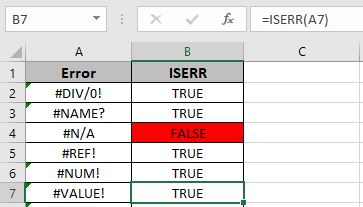Excel #REF Error
The 'ref' in #REF stands for reference. This error occurs when a formula refers to a location that does not exist. This happens when we delete cells from ranges to which a formula refers too.

In the below gif, the sum formula refers to A2 and B2. When I delete A2, the formula turns into a #REF error.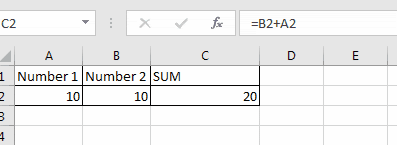Solve Excel #REF error:
The best thing is to be careful before deleting cells in data. Make sure that no formula refers to that cell.

If you have #REF error already then trace then delete it from the formula.

For example, once you get a #REF error, your formula will look like this.

=A2+#REF!

You can just remove the #REF! From the formula to get an error-free formula. If you want to do it in bulk then use find and replace feature. Press CTRL+H to open find and replace. In find box, write #REF. Leave replace box empty. Hit Replace All button.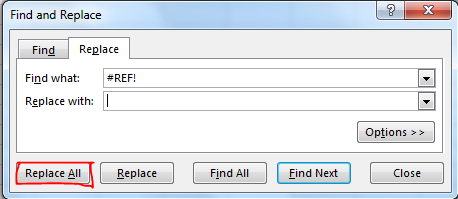If you want to readjust the reference to a new cell then do it manually and replace the #REF! With that valid reference.

Excel #NAME Error
The #NAME occurs in excel when it can't identify a text in a formula. For example, if you misspell a function's name, excel will show the #NAME error. If a formula refers to a name that does not exist on the sheet, it will show #NAME error.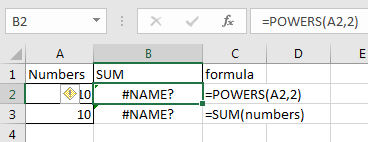In the above image, the cell B2 has formula =POWERS(A2,2). POWERS is not a valid function in excel, hence it returns a #NAME error.

In cell B3 we have =SUM(numbers). The SUM is a valid function of excel but "numbers" named range does not exist on sheet. Hence excel returns #NAME? Error.

How to avoid #NAME error in excel?

To avoid #NAME error in excel always spell function names correctly. You can use excel suggestions to be sure that you are using a valid function. Whenever we type characters after the equals sign, excel show functions and named ranges on the sheet, starting from that character/s. Scroll down to function name or range name in the suggestion list press tab to use that function.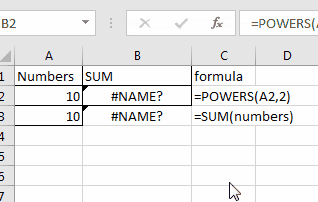Excel #DIV/0! Error
As the name suggests, this error occurs when a formula results in division by zero. This error may also occur when you delete some value from a cell on which a division formula is dependent.

How to solve #DIV/0! Error.

This can be easily solved by being careful with data and putting a check if a formula will result in #DIV/0! error. Here is a sample formula to do so.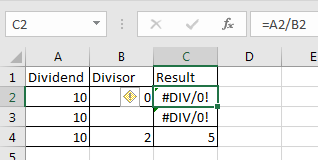=IF(B2=0, A2, A2/B2)

We will have DIV/0 error only if the divisor is 0 or blank. Hence we check the divisor (B2), if its a zero then print A2 else divide A2 with B2. This will work for blank cells too.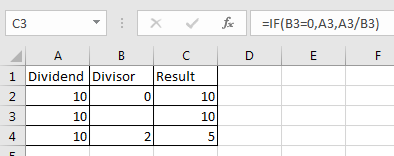Excel #NUM Error

This error occurs when a number can't be displayed on the screen. The reason can be that the number is too small or too big to be displayed. Another reason could be that a calculation can't be performed with given number.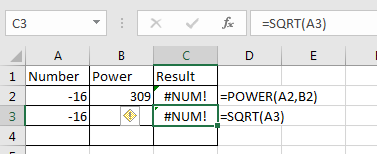=SQRT(ABS(A3))

This will return 4. If you want to get the negative value then use the negative sign before the function. Then of course you can use error handling function of course.
On solving #NUM error we have a dedicated article. You can check it here.

#NULL Error in Excel
This is a rare type of error. #NULL error caused by incorrect cell referencing. For example if you want to give reference of a range A2:A5 in SUM function, but by mistake you type A2 A5. This will generate #NULL error. As you can see in below image, the formula returns #NULL error.
If you replace space with column (:) then the #NULL error will be gone and you'll get sum of the A2:A5. If you replace space with comma (,) , you will get sum of A2 and A5.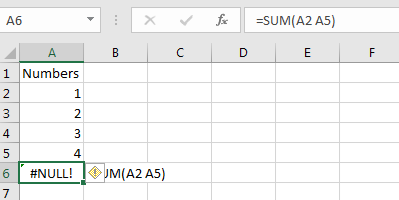How to solve #NULL error?

As we know that the #NULL error is caused by typo. To avoid it, try to select ranges using curser instead of typing it manually.

There will be times when you have to type range address from keyboard. Always take care of the connecting symbol column (:) or comma (,) to avoid.

If you have existing #NULL error then check the references. Most probably you have missed column (:) or comma (,) between two cell references. Replace them with appropriate symbol and you are good to go.
###### Error in Excel
Sometimes we think that this error is caused due to insufficient space to show a value, but this is not the case. In this case you can just extend the width of the cell to view the value in cell. I won't call it an error.
In fact, this error caused when we try to show a negative time value (there no such thing as negative time). For example, if we try to subtract 1 from 12:21:00 PM it will return ######. In excel the first date is 1/1/1900 00:00. If you try to subtract time that goes before that, excel will show you ###### error. The more you expand the width the more # you will get. I have elaborated it in this article.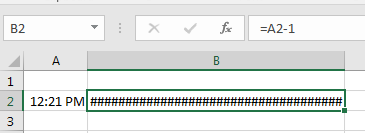How to avoid ###### Excel error?
Before doing arithmetic calculations in excel with time value keep these things in mind.

• The minimum time value is  1/1/1900 00:00. You can't have a valid date before this in excel
• 1 is equal to 24 hours (1 day) in excel. While subtracting hours, minutes and seconds, convert them into equivalent values. For example, to subtract 1 hour from 12:21 PM, you need to subtract 1/24 from it.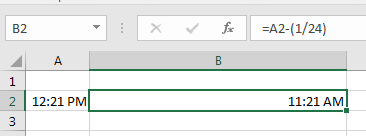Tracking Down Errors in Excel
Now we know what common excel formulas are and why they occur. We also discussed what possible solution can be for each type of excel errors.
Sometimes in excel reports, we get errors and we couldn't know from where the error is actually occuring. It gets hard to solve these kind of errors. To track down these errors excel provides error tracing functionality in formula tab.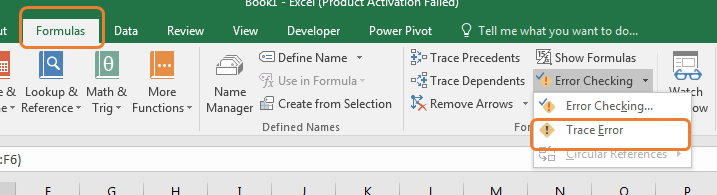I have discussed error tracing in excel in detail.

Solving Logical Errors in Formula

Logical errors are tough to find and resolve. No massage shown by excel when you have a logical error in your function. Everything looks fine. But only you know that there something is wrong there. For example, while getting percentage of a part of whole, you would divide part by total (=(part/total)*100). It should be always equal or less then 100%. When you get more than 100%, you know something is wrong.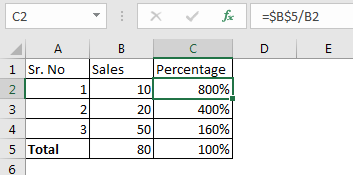This was a simple logical error. But sometimes your data comes from several sources, in that case it gets hard to solve logical problems. One way is to evaluate your formula.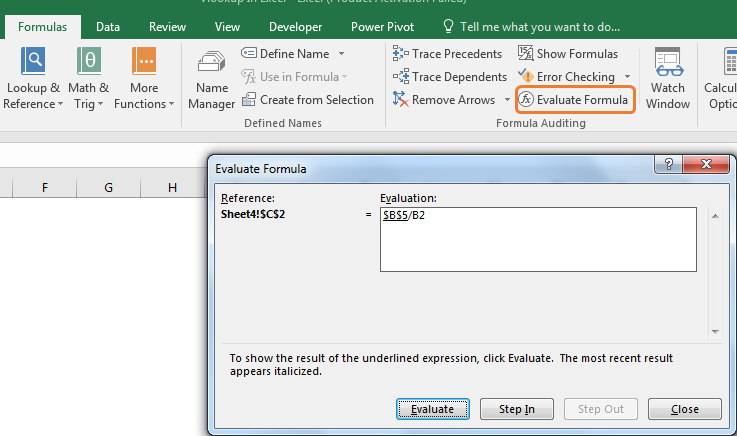In formula tab, evaluate formula option is available. Select you formula and click on it. Each step of your calculation will be show to you that leads to your final result. Here you can check where the problem has occurred or where the calculation has gone wrong.

You can also yous trace dependents and precedents to see on which references your formula depends and what formulas depends on a perticular cell.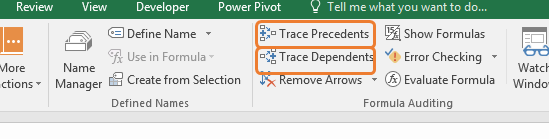So yeah guys, these were some common error types every excel user faces. We learned why each type of error occurs and how can we avoid them. We learned about dedicated error handling excel function too. In this article, we have links to related pages that discuss the problem in detail. You can check them out. If you have any specific type of error that is annoying you, mention it in the comments section below. You will get the solution sortly.

Related Articles:

How to correct a #NUM! Error

Create Custom Error Bars In Excel 2016

#VALUE Error And How to Fix It in Excel

How to Trace and Fix Formula Errors in Excel

Popular Articles:

The VLOOKUP Function in Excel

COUNTIF in Excel 2016

How to Use SUMIF Function in Excel

Terms and Conditions of use

The applications/code on this site are distributed as is and without warranties or liability. In no event shall the owner of the copyrights, or the authors of the applications/code be liable for any loss of profit, any problems or any damage resulting from the use or evaluation of the applications/code.